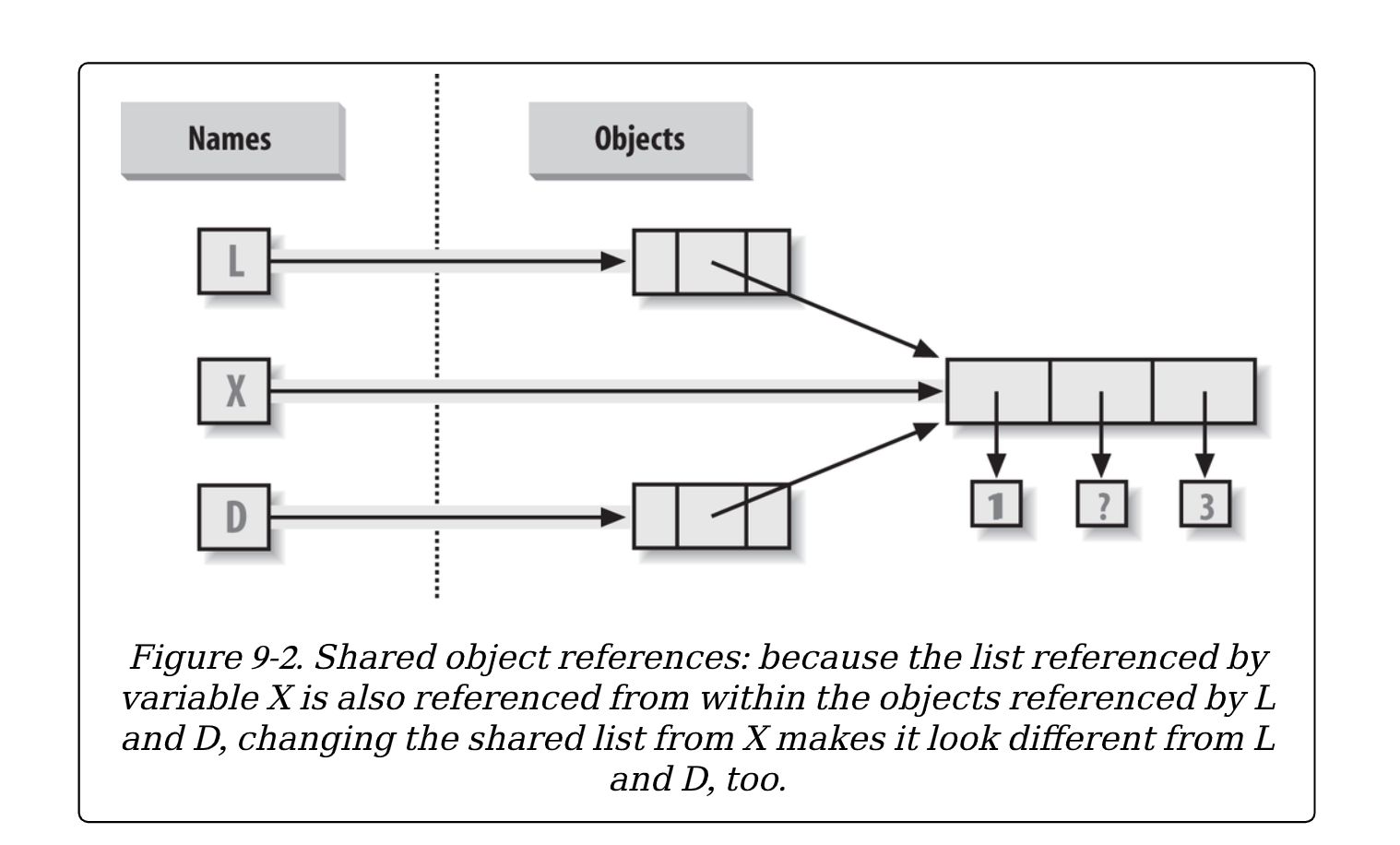# 核心对象类型复习和总结——引用 VS 拷贝

``````>>> X = [1, 2, 3]
>>> L = ['a', X, 'b'] # Embed references to X's object
>>> D = {'x':X, 'y':2}````````````>>> X = 'surprise' # Changes all three references!
>>> L
['a', [1, 'surprise', 3], 'b']
>>> D
{'x': [1, 'surprise', 3], 'y': 2}``````

• 限制为空的切片操作（L[:]）拷贝序列。
• 字典、set和列表的`copy`方法（X.copy()）拷贝字典、set或列表（列表的`copy`是从3.3版本开始新增的）。
• 一些内置函数，如`list``dict`创建拷贝（`list(L)`, `dict(D)`, `set(S)`）。
• 当需要时，`copy`标准库模块创建完整拷贝。

``````>>> L = [1,2,3]
>>> D = {'a':1, 'b':2}``````

``````>>> A = L[:] # Instead of A = L (or list(L) 也行)
>>> B = D.copy() # Instead of B = D (ditto for sets)``````

``````>>> A = 'Ni'
>>> B['c'] = 'spam'
>>>
>>> L, D
([1, 2, 3], {'a': 1, 'b': 2})
>>> A, B
([1, 'Ni', 3], {'a': 1, 'c': 'spam', 'b': 2})``````

``````>>> X = [1, 2, 3]
>>> L = ['a', X[:], 'b'] # Embed copies of X's object
>>> D = {'x':X[:], 'y':2}
>>> X = 'surprise'
>>> L
['a', [1, 2, 3], 'b']
>>> D
{'x': [1, 2, 3], 'y': 2}``````

``````import copy
X = copy.deepcopy(Y) # Fully copy an arbitrarily nested object
Y``````